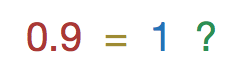# Can $< 1 = 1$?Does there exists intervals $I_1, I_2, \ldots$, each of which are subintervals of $[ 0,1]$, such that
1. the total length of these subintervals is strictly less than 1.
2. For each rational number $r \in [0,1]$, there exists a $j$ such that $r \in I_j$.Note by Calvin Lin
6 years, 10 months ago

This discussion board is a place to discuss our Daily Challenges and the math and science related to those challenges. Explanations are more than just a solution — they should explain the steps and thinking strategies that you used to obtain the solution. Comments should further the discussion of math and science.

When posting on Brilliant:

• Use the emojis to react to an explanation, whether you're congratulating a job well done , or just really confused .
• Ask specific questions about the challenge or the steps in somebody's explanation. Well-posed questions can add a lot to the discussion, but posting "I don't understand!" doesn't help anyone.
• Try to contribute something new to the discussion, whether it is an extension, generalization or other idea related to the challenge.
• Stay on topic — we're all here to learn more about math and science, not to hear about your favorite get-rich-quick scheme or current world events.

MarkdownAppears as
*italics* or _italics_ italics
**bold** or __bold__ bold
- bulleted- list
• bulleted
• list
1. numbered2. list
1. numbered
2. list
Note: you must add a full line of space before and after lists for them to show up correctly
paragraph 1paragraph 2

paragraph 1

paragraph 2

[example link](https://brilliant.org)example link
> This is a quote
This is a quote
    # I indented these lines
# 4 spaces, and now they show
# up as a code block.

print "hello world"
# I indented these lines
# 4 spaces, and now they show
# up as a code block.

print "hello world"
MathAppears as
Remember to wrap math in $$ ... $$ or $ ... $ to ensure proper formatting.
2 \times 3 $2 \times 3$
2^{34} $2^{34}$
a_{i-1} $a_{i-1}$
\frac{2}{3} $\frac{2}{3}$
\sqrt{2} $\sqrt{2}$
\sum_{i=1}^3 $\sum_{i=1}^3$
\sin \theta $\sin \theta$
\boxed{123} $\boxed{123}$

## Comments

Sort by:

Top Newest

We can write the set of rational numbers in $[0,1]$ as $\{m/n : 0\leq m \leq n, n \geq 1\}$. Now exclude the point $1$ from all these sets and enclose each rational point with denominator $n$ by a sub interval of length $3^{-n}$, lying completely in $[0,1]$ (e.g. enclose the point $m/n$ by the sub-interval $[m/n, m/n + 3^{-n}]$). The total length of all these sub-intervals is $\sum_{n=1}^{\infty} n3^{-n}=\frac{3}{4}$. Finally enclose the point $1$ by the sub-interval $[9/10,1]$. Hence all rational points are enclosed and total length of the sub-intervals = $3/4+1/10 <1$.

- 6 years, 10 months ago

Log in to reply

This was really good.

- 6 years, 10 months ago

Log in to reply

Quick note that the total length is less than $17/20$ because of overlapping intervals.

- 6 years, 10 months ago

Log in to reply

Indeed, the rationals are measure-zero and countable (despite being dense in $\mathbb{R}$); they are a null set. The total length of the required subintervals can be any $\epsilon > 0$.

- 6 years, 10 months ago

Log in to reply

That does not prove existence of sub intervals of the required properties.

- 6 years, 10 months ago

Log in to reply

The proof of my initial statement eludes me. A proof that the total length is less than $\epsilon$ for any $\epsilon>0$ is much easier (order the rationals and construct intervals around them with lengths of the terms of a converging infinite geometric series of your choice).

If anyone is able to prove my original statement (especially constructively), by all means, post it here. The "overlaps" of the intervals are bugging me quite a bit.

- 6 years, 10 months ago

Log in to reply

0.999999999999999.................................=1

- 6 years, 10 months ago

Log in to reply

×

Problem Loading...

Note Loading...

Set Loading...